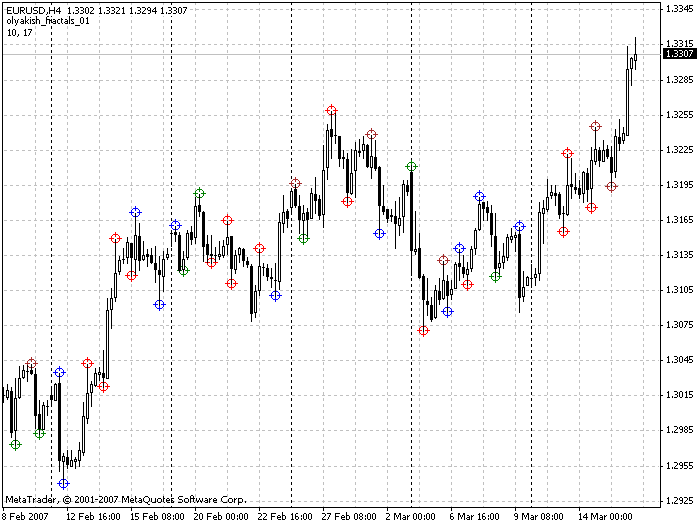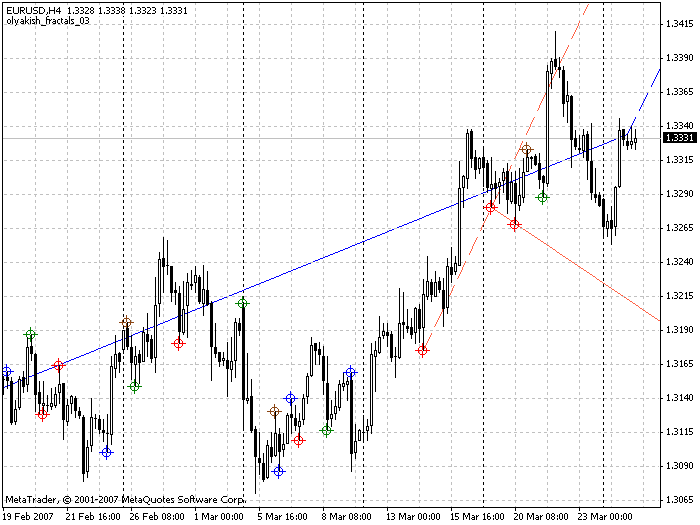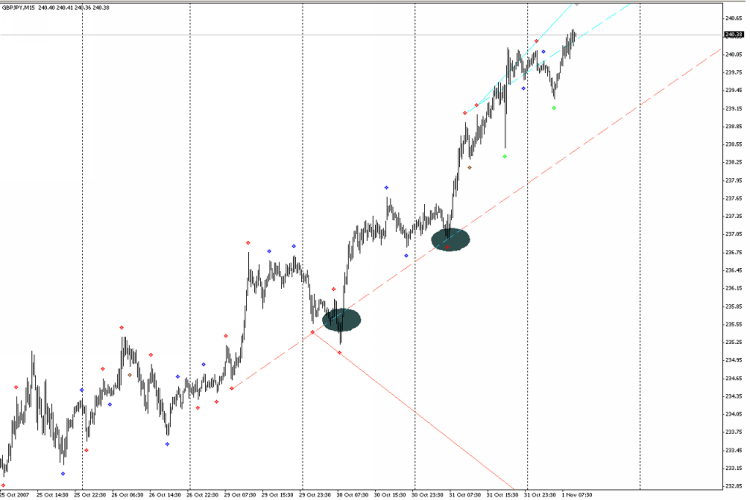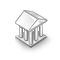Interesting script?
So post a link to it -
let others appraise it
You liked the script? Try it in the MetaTrader 5 terminal# New_Fractal_Lines - indicator for MetaTrader 4

|
Views:
23429
Rating:
(12)
Published:
2016.03.25 13:45
Updated:
2016.04.21 11:55

After reading the first "Chaos" by B. Williams I came up with the idea to implement not the "squat" bar, but the "squat" fractal. The idea: take the two nearby divergent fractals. Let the first (further in history) is downwards, the second (closer in history) is upwards. Calculate the delta of the fractal:
(High[i+2] - Low[i+2] + High[i+1] - Low[i+1] + High[i] - Low[i] + High[i-1] - Low[i-1] + High[i-2] - Low[i-2]);

Calculate the total (tick) volume of the fractal:
(Volume[i+2] + Volume[i+1] + Volume[i] + Volume[i-1] + Volume[i-2]);

Calculate the MFI of the fractal:
(delta / total volume);

The same is done with the second fractal. Next, if

`(MFI1 > MFI2  && Vol1 < Vol2) == true;`

then draw a dot above the High of this fractal. The resistance line is the trend line plotted across two adjacent upward "squat" fractals. Almost the same is with a pair of fractals in different directions: first up, second down. The only difference is that the dot is drawn lower than the Low below the downward "squat" fractal. The resistance line is the trend line plotted across two adjacent downward "squat" fractals.

The trading system using this indicator is not fully developed, but I think:
- If the support line (brown) is directed upward and the price is above it, wait for the price to close below this line and sell;
- If the support line (brown) is directed downward and the price breaks (closes) above it, then do not enter the trade.

Exactly the opposite is true for trading the support line breakouts. But this is not the final version.

Feel free to comment on the improvement of both the indicator and the trading system based on it.

15.03.2007.
Added the olyakish_fractals_01.mq4 indicator, that displays other fractals as well, namely "green", "squat", "false" and "fading".22.03.2007.
Added the new version of the olyakish_fractals_02.mq4 indicator. It implements the fractal filter by their inner volume (as a percentage). As a result, the chart will have fewer fractals, the most significant ones will be left.

- trade the breakout of the red fractals (squat), it is also possible to trade in the opposite direction simultaneously, as soon as the blue (false) fractals appears with the StopLoss on the current fractal. Thus, it is possible to take from a few pips to a solid movement.

27.03.2007.
New version of the olyakish_fractals_03.mq4 indicator. Added plotting of the current support/resistance lines and the ability to enable/disable the plotting of the previous lines. This indicator is quite dependent on the tick volume. As a solution to this situation - use the quotes as close to real as possible (with no server filtering).01.11.2007 added the ability to choose the number of bars in a fractal;
it is possible to select the fractal type for drawing the support/resistance lines
Usage: I think, if the support line is directed upwards and the price touched it and bounced back, or broke it for a short time, and closed above the specified line, then the rising movement is likely to resume (trade by trend)
the same applies to resistance line.26.11.2007 Fixed some bugs in the version 06а.

Translated from Russian by MetaQuotes Ltd.
Original code: https://www.mql5.com/ru/code/7073Function library for maintaining positions with a simple trailing stop

With the help of this library it is possible to implement the ability to maintain positions with a simple trailing stop in any Expert Advisor.Library of functions for working with INI-files.

The library contains five functions for reading the values from an INI-file and five functions for writing the values to an INI-file. Thus, there are five pairs of functions to read/write the values of 'integer array', 'boolean', 'double', 'integer' and 'string' types.WATR trend indicator, simple but clear

A simple trend indicator that clearly shows the direction of the current trend. It can be used in the breakout trading systems.Comment2

The Comment2 library expands the capabilities of the standard Comment function for comment output.